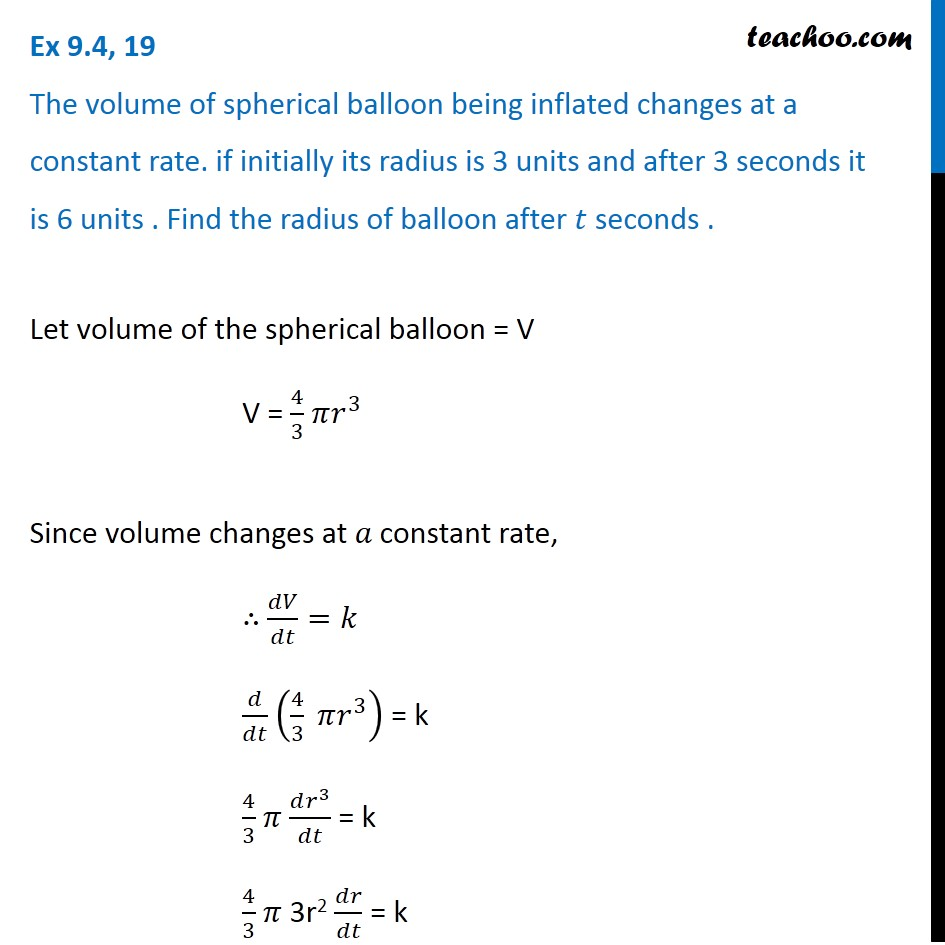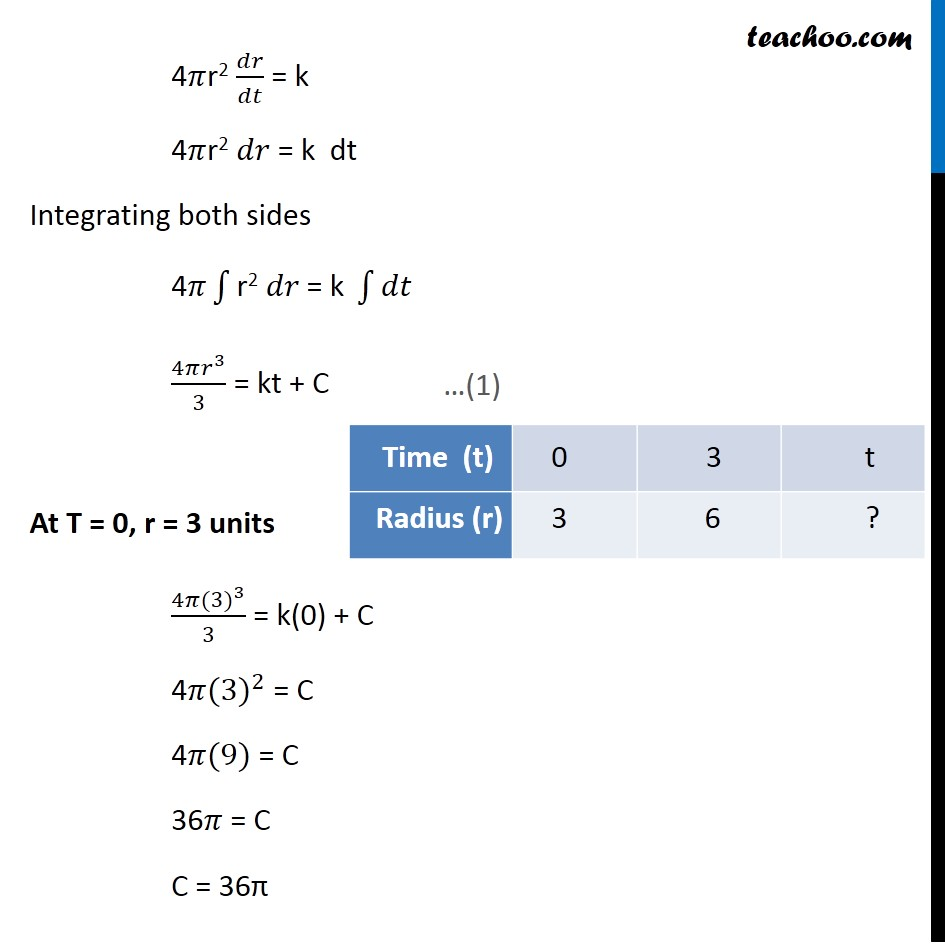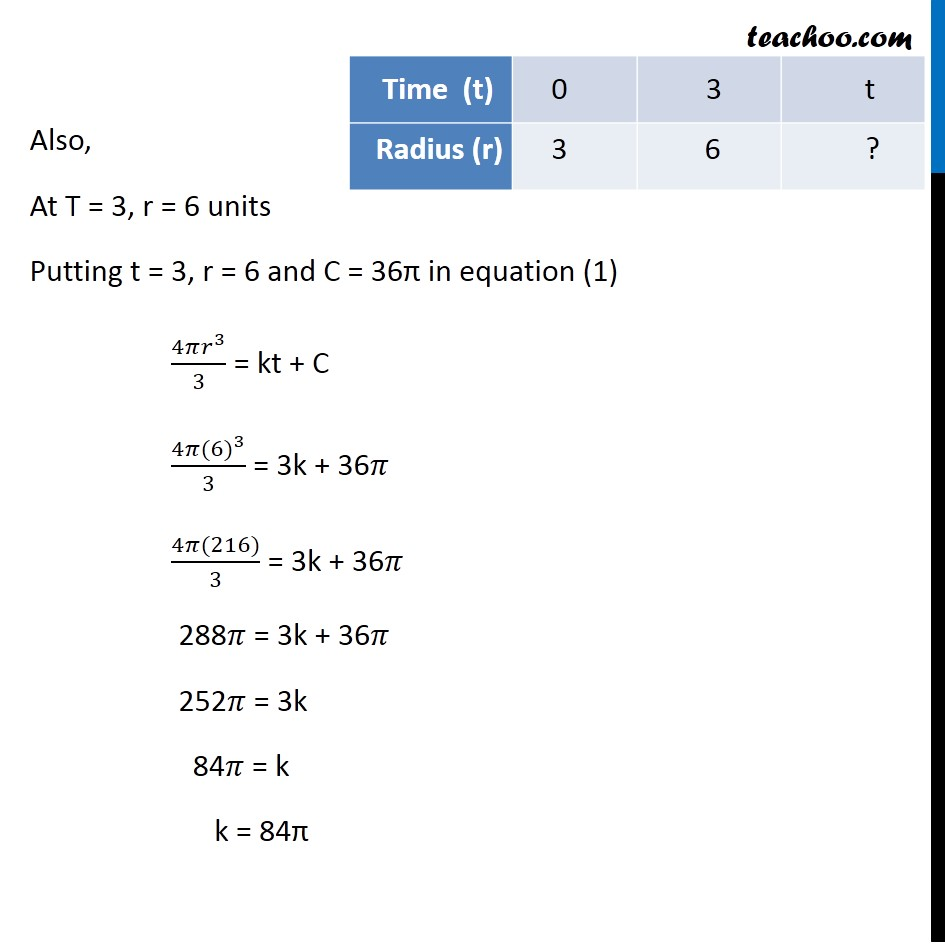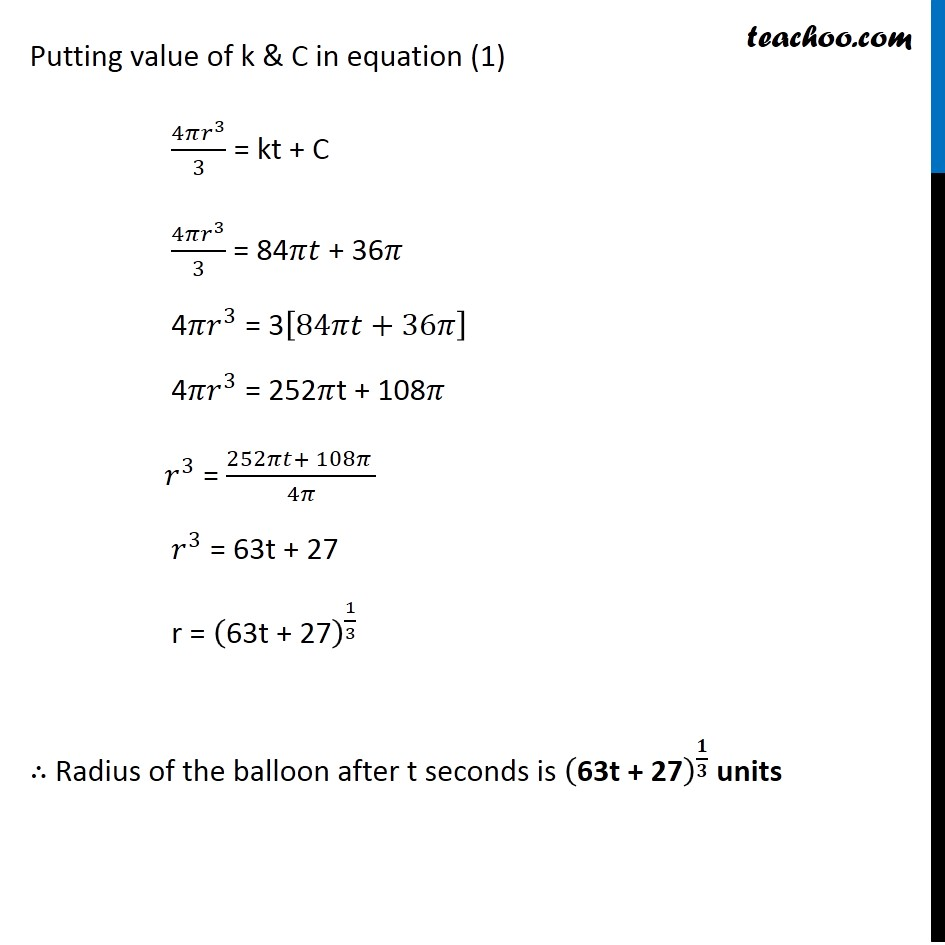Ex 9.3

Chapter 9 Class 12 Differential Equations
Serial order wiseLearn in your speed, with individual attention - Teachoo Maths 1-on-1 Class

### Transcript

Ex 9.3, 19 The volume of spherical balloon being inflated changes at a constant rate. if initially its radius is 3 units and after 3 seconds it is 6 units . Find the radius of balloon after 𝑡 seconds . Let volume of the spherical balloon = V V = 4/3 𝜋𝑟^3 Since volume changes at 𝑎 constant rate, ∴ 𝑑𝑉/𝑑𝑡=𝑘 𝑑/𝑑𝑡 (4/3 " " 𝜋𝑟^3 ) = k 4/3 𝜋 (𝑑𝑟^3)/𝑑𝑡 = k 4/3 𝜋 3r2 𝑑𝑟/𝑑𝑡 = k 4𝜋r2 𝑑𝑟/𝑑𝑡 = k 4𝜋r2 𝑑𝑟 = k dt Integrating both sides 4𝜋∫1▒〖"r2 " 𝑑𝑟" = k " 〗 ∫1▒𝑑𝑡 (4𝜋𝑟^3)/3 = kt + C At T = 0, r = 3 units 〖4𝜋(3)〗^3/3 = k(0) + C 〖"4" 𝜋(3)〗^2 = C "4" 𝜋(9) = C 36𝜋 = C C = 36π …(1) Also, At T = 3, r = 6 units Putting t = 3, r = 6 and C = 36π in equation (1) (4𝜋𝑟^3)/3 = kt + C 〖4𝜋(6)〗^3/3 = 3k + 36𝜋 (4𝜋(216))/3 = 3k + 36𝜋 288𝜋 = "3k + 36" 𝜋 252𝜋 = "3k " 84𝜋 = "k" k = 84π Putting value of k & C in equation (1) (4𝜋𝑟^3)/3 = kt + C 〖4𝜋𝑟〗^3/3 = 84𝜋𝑡 + 36𝜋 〖"4" 𝜋𝑟〗^3 = 3[84𝜋𝑡+36𝜋] 〖"4" 𝜋𝑟〗^3 = 252𝜋t + 108𝜋 𝑟^3 = (252𝜋𝑡+ 108𝜋" " )/4𝜋 𝑟^3 = 63t + 27 r = ("63t + 27" )^(1/3) ∴ Radius of the balloon after t seconds is ("63t + 27" )^(𝟏/𝟑) units